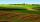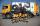# Equations - simple

Solve system of linear equations:

x-2y=6
3x+2y=4

Result

x =  2.5
y =  -1.75

#### Solution:

x-2y=6
3x+2y=4

x-2y = 6
3x+2y = 4

x = 52 = 2.5
y = -74 = -1.75

Calculated by our linear equations calculator.

Leave us a comment of example and its solution (i.e. if it is still somewhat unclear...):

Showing 1 comment:Math student
hi i liked this site it helps me in practicing for my exams and knowledge#### To solve this example are needed these knowledge from mathematics:

Do you have a system of equations and looking for calculator system of linear equations?

## Next similar examples:

1. EquationsSolve following system of equations: 6(x+7)+4(y-5)=12 2(x+y)-3(-2x+4y)=-44
2. Linear systemSolve this linear system (two linear equations with two unknowns): x+y =36 19x+22y=720
3. Two numbersWe have two numbers. Their sum is 140. One-fifth of the first number is equal to half the second number. Determine those unknown numbers.
4. TheatroTheatrical performance was attended by 480 spectators. Women were in the audience 40 more than men and children 60 less than half of adult spectators. How many men, women and children attended a theater performance?
5. SolutionsHow much 60% solution and how much 35% solution is needed to create 100 l of 40% solution?
6. Three friendsThe three friends spent 600 KC in a teahouse. Thomas paid twice as much as Paul. Paul a half less than Zdeněk. How many each paid?
7. Land areasTwo land areas is 244 m2. The first parcel is 40 m2 less than twice of the second one. What have acreage of each parcel?
8. Three brothersThe three brothers have a total of 42 years. Jan is five years younger than Peter and Peter is 2 years younger than Michael. How many years has each of them?
9. MushroomsEva and Jane collected 114 mushrooms together. Eve found twice as much as Jane. How many mushrooms found each of them?
10. TreesAlong the road were planted 250 trees of two types. Cherry for 60 CZK apiece and apple 50 CZK apiece. The entire plantation cost 12,800 CZK. How many was cherries and apples?
11. Hotel roomsIn the 45 rooms, there were 169 guests, some rooms were three-bedrooms and some five-bedrooms. How many rooms were?
12. Football seasonDalibor and Adam together scored 97 goals in the season. Adam scored 9 goals more than Dalibor. How many goals scored each?
13. Stamps 2Dennis spent 34.15 on stamps. The number of .56 is 10 less than four times of stamps bought for .41. How many of each stamp did he buy?
14. Three figures - numbersThe sum of three numbers, if each is 10% larger than the previous one, is 662. Determine the figures.
15. Factory and divisionsThe factory consists of three auxiliary divisions total 2,406 employees. The second division has 76 employees less than 1st division and 3rd division has 212 employees more than the 2nd. How many employees has each division?
16. Collecting dayAt collecting day at school pupils of three classes took total of 1063 kg of paper. Class B collected half more than A Class and class C 55 kg more than B. How many kg of paper collected each class?
17. Vijuviju has 40 chickens and rabbits. If in all there are 90 legs. How many rabbits are there with viju??